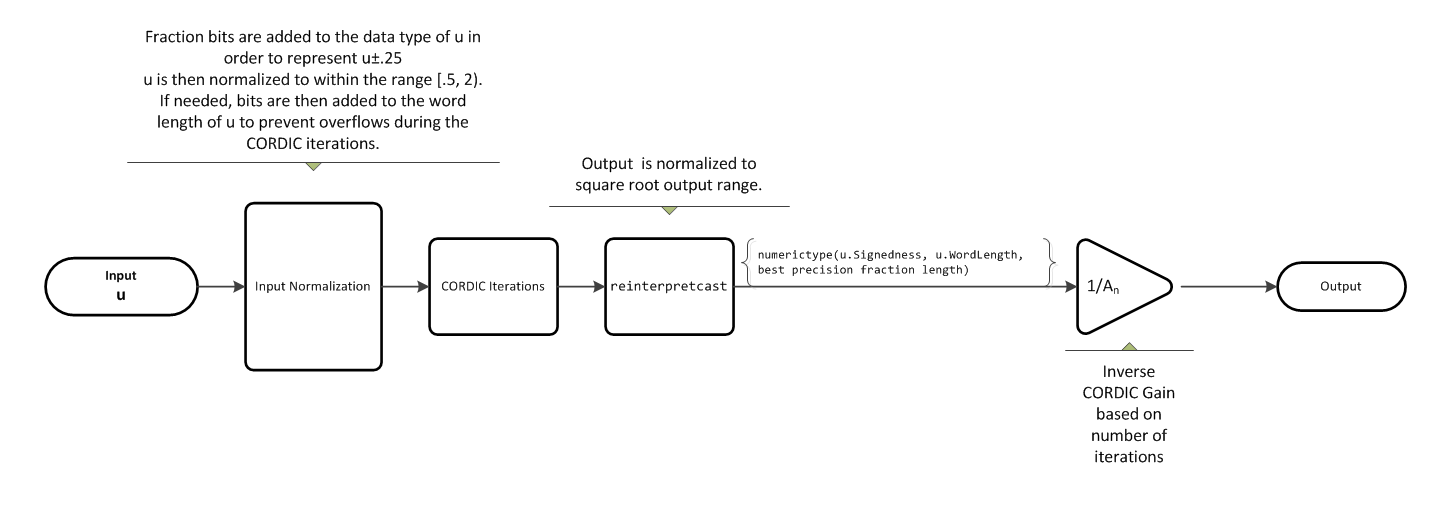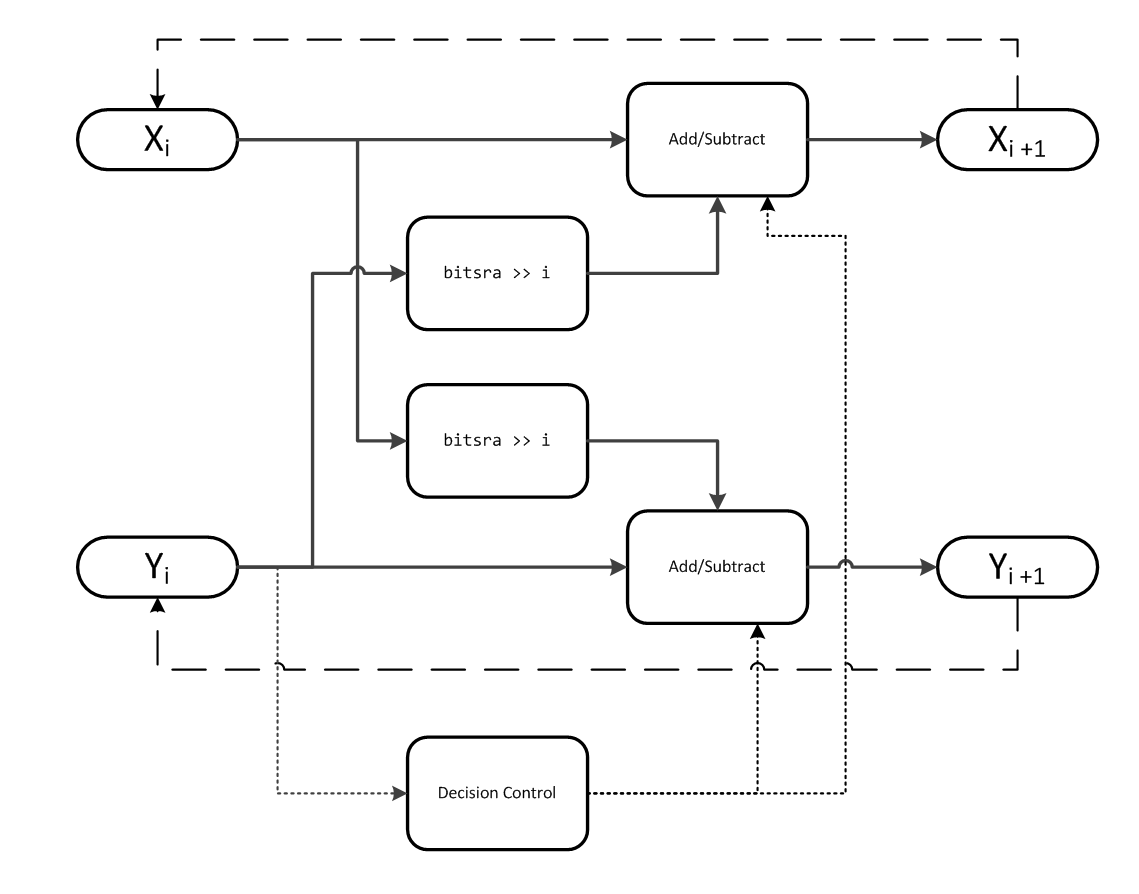# cordicsqrt

CORDIC-based approximation of square root

## Syntax

``y=cordicsqrt(u)``
``y=cordicsqrt(u, niters)``
``````y=cordicsqrt(___, 'ScaleOutput', B)``````

## Description

example

````y=cordicsqrt(u)` computes the square root of `u` using a CORDIC algorithm implementation. ```

example

````y=cordicsqrt(u, niters)` computes the square root of `u` by performing `niters` iterations of the CORDIC algorithm.```

example

``````y=cordicsqrt(___, 'ScaleOutput', B)``` scales the output depending on the Boolean value of `B`.```

## Examples

collapse all

Find the square root of `fi` object `x` using a CORDIC implementation.

```x = fi(1.6,1,12); y = cordicsqrt(x)```
```y = 1.2646 DataTypeMode: Fixed-point: binary point scaling Signedness: Signed WordLength: 12 FractionLength: 10 ```

Because you did not specify `niters`, the function performs the maximum number of iterations, `x.WordLength - 1`.

Compute the difference between the results of the `cordicsqrt` function and the double-precision `sqrt` function.

`err = abs(sqrt(double(x))-double(y))`
```err = 1.0821e-04 ```

Compute the square root of x with three iterations of the CORDIC kernel.

```x = fi(1.6,1,12); y = cordicsqrt(x,3)```
```y = 1.2646 DataTypeMode: Fixed-point: binary point scaling Signedness: Signed WordLength: 12 FractionLength: 10 ```

Compute the difference between the results of the `cordicsqrt` function and the double-precision `sqrt` function.

`err = abs(sqrt(double(x))-double(y))`
```err = 1.0821e-04 ```
```x = fi(1.6,1,12); y = cordicsqrt(x, 'ScaleOutput', 0)```
```y = 1.0479 DataTypeMode: Fixed-point: binary point scaling Signedness: Signed WordLength: 12 FractionLength: 10 ```

The output, `y`, was not scaled by the inverse CORDIC gain factor.

Compare the results produced by 10 iterations of the `cordicsqrt` algorithm to the results of the double-precision `sqrt` function.

```% Create 500 points between [0, 2) stepSize = 2/500; XDbl = 0:stepSize:2; XFxp = fi(XDbl, 1, 12); % signed, 12-bit fixed-point sqrtXRef = sqrt(double(XFxp)); % reference results % Use 12-bit quantized inputs and set the number % of iterations to 10. % Compare the fixed-point CORDIC results to the % double-precision sqrt function results. niters = 10; cdcSqrtX = cordicsqrt(XFxp, niters); errCdcRef = sqrtXRef - double(cdcSqrtX); figure hold on axis([0 2 -.5 1.5]) plot(XFxp, sqrtXRef, 'b') plot(XFxp, cdcSqrtX, 'g') plot(XFxp, errCdcRef, 'r') ylabel('Sqrt(x)') gca.XTick = 0:0.25:2; gca.XTickLabel = {'0','0.25','0.5','0.75','1','1.25','1.5','1.75','2'}; gca.YTick = -.5:.25:1.5; gca.YTickLabel = {'-0.5','-0.25','0','0.25','0.5','0.75','1','1.25','1.5'}; ref_str = 'Reference: sqrt(double(X))'; cdc_str = sprintf('12-bit CORDIC square root; N = %d', niters); err_str = sprintf('Error (max = %f)', max(abs(errCdcRef))); legend(ref_str, cdc_str, err_str, 'Location', 'southeast')```## Input Arguments

collapse all

Data input array, specified as a positive scalar, vector, matrix, or multidimensional array of fixed-point or built-in data types. When the input array contains values between 0.5 and 2, the algorithm is most accurate. A pre- and post-normalization process is performed on input values outside of this range. For more information on this process, see Pre- and Post-Normalization.

Data Types: `fi`|`single` | `double` | `int8` | `int16` | `int32` | `int64` | `uint8` | `uint16` | `uint32` | `uint64`

The number of iterations that the CORDIC algorithm performs, specified as a positive, integer-valued scalar. If you do not specify `niters`, the algorithm uses a default value. For fixed-point inputs, the default value of `niters` is `u.WordLength - 1`. For floating-point inputs, the default value of `niters` is 52 for double precision; 23 for single precision.

Data Types: `fi`|`single` | `double` | `int8` | `int16` | `int32` | `int64` | `uint8` | `uint16` | `uint32` | `uint64`

Boolean value that specifies whether to scale the output by the inverse CORDIC gain factor. If you set `ScaleOutput` to `true` or `1`, the output values are multiplied by a constant, which incurs extra computations. If you set `ScaleOutput` to `false` or `0`, the output is not scaled.

Data Types: `logical`

## Output Arguments

collapse all

Output array, returned as a scalar, vector, matrix, or multidimensional array.

collapse all

### CORDIC

CORDIC is an acronym for COordinate Rotation DIgital Computer. The Givens rotation-based CORDIC algorithm is one of the most hardware-efficient algorithms available because it requires only iterative shift-add operations (see References). The CORDIC algorithm eliminates the need for explicit multipliers. Using CORDIC, you can calculate various functions such as sine, cosine, arc sine, arc cosine, arc tangent, and vector magnitude. You can also use this algorithm for divide, square root, hyperbolic, and logarithmic functions.

Increasing the number of CORDIC iterations can produce more accurate results, but doing so increases the expense of the computation and adds latency.

## Algorithms

collapse all

### Signal Flow DiagramsFor further details on the pre- and post-normalization process, see Pre- and Post-Normalization.

CORDIC Hyperbolic KernelX is initialized to `u'+.25`, and Y is initialized to `u'-.25`, where `u'` is the normalized function input.

With repeated iterations of the CORDIC hyperbolic kernel, X approaches ${A}_{N}\sqrt{u\text{'}}$, where AN represents the CORDIC gain. Y approaches `0`.

### Pre- and Post-Normalization

For input values outside of the range of [0.5, 2) a pre- and post-normalization process occurs. This process performs bitshifts on the input array before passing it to the CORDIC kernel. The result is then shifted back into the correct output range during the post-normalization stage. For more details on this process see “Overcoming Algorithm Input Range Limitations” in Compute Square Root Using CORDIC.

### fimath Propagation Rules

CORDIC functions discard any local `fimath` attached to the input.

The CORDIC functions use their own internal `fimath` when performing calculations:

• `OverflowAction``Wrap`

• `RoundingMethod``Floor`

The output has no attached `fimath`.

 Volder, JE. “The CORDIC Trigonometric Computing Technique.” IRE Transactions on Electronic Computers. Vol. EC-8, September 1959, pp. 330–334.

 Andraka, R. “A survey of CORDIC algorithm for FPGA based computers.” Proceedings of the 1998 ACM/SIGDA sixth international symposium on Field programmable gate arrays. Feb. 22–24, 1998, pp. 191–200.

 Walther, J.S. “A Unified Algorithm for Elementary Functions.” Hewlett-Packard Company, Palo Alto. Spring Joint Computer Conference, 1971, pp. 379–386. (from the collection of the Computer History Museum). www.computer.org/csdl/proceedings/afips/1971/5077/00/50770379.pdf

 Schelin, Charles W. “Calculator Function Approximation.” The American Mathematical Monthly. Vol. 90, No. 5, May 1983, pp. 317–325.